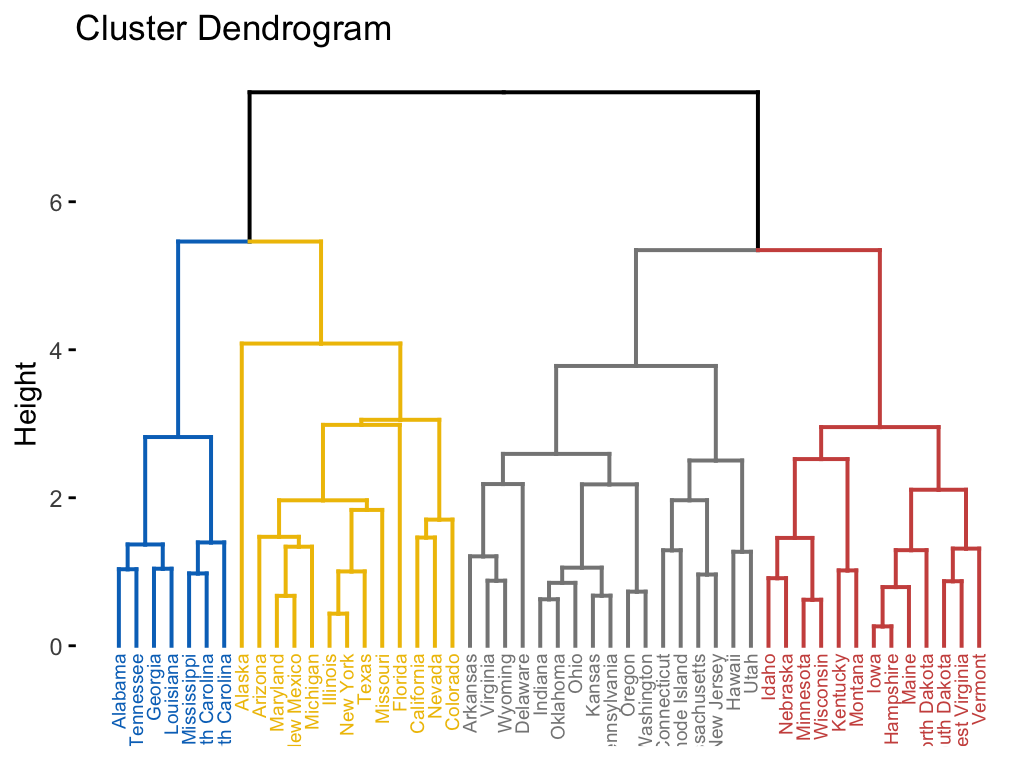# Hierarchical Clustering in R: The Essentials

## Divisive Hierarchical Clustering

The divisive hierarchical clustering, also known as DIANA (DIvisive ANAlysis) is the inverse of agglomerative clustering .

This article introduces the divisive clustering algorithms and provides practical examples showing how to compute divise clustering using R.

#### Related Book

Practical Guide to Cluster Analysis in R

## Algorithm

It starts by including all objects in a single large cluster. At each step of iteration, the most heterogeneous cluster is divided into two. The process is iterated until all objects are in their own cluster.

Recall that, divisive clustering is good at identifying large clusters while agglomerative clustering is good at identifying small clusters.

## Computation

The R function diana() [cluster package] can be used to compute divisive clustering. It returns an object of class “diana” (see ?diana.object) which has also methods for the functions: print(), summary(), plot(), pltree(), as.dendrogram(), as.hclust() and cutree().

The output of DIANA can be visualized as dendrograms using the function fviz_dend() [factoextra package]. For example, the following R code shows how to computes and visualize divise clustering:

# Compute diana()
library(cluster)
res.diana <- diana(USArrests, stand = TRUE)

# Plot the dendrogram
library(factoextra)
fviz_dend(res.diana, cex = 0.5,
k = 4, # Cut in four groups
palette = "jco" # Color palette
)For interpreting dendrograms, read the “agglomerative clustering” chapter.